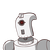# 8Rahul horrowed & 1, 200 from his friend at thegate of 12% pa find the amount to be paid atthe end of 3 year

8
Rahul horrowed & 1, 200 from his friend at the
gate of 12% pa find the amount to be paid at
the end of 3 years​

### 1 thought on “8<br />Rahul horrowed & 1, 200 from his friend at the<br />gate of 12% pa find the amount to be paid at<br />the end of 3 year”

1.We have Principal as ₹1200 and Rate of 12% and time taken for borrow is 3 years.

we know that:

$$\circ \ {\purple{\underline{\boxed{\sf{ Interest_{(Simple)} = \dfrac{PRT}{100} }}}}} \\ \\ \\ \colon\implies{\sf{ \dfrac{12 \cancel{00} \times 12 \times 3}{ \cancel{100} } }} \\ \\ \\ \colon\implies{\sf{ 12 \times 12 \times 3 }} \\ \\ \\ \colon\implies{\sf{ Rs. \ 432 }} \\$$

~Now, we’ve to find the Amount paid after 3 years as:-

$$\circ \ {\red{\underline{\boxed{\sf{ Amount = Principal+Interest }}}}} \\ \\ \colon\mapsto{\sf{ 1200 + 432 }} \\ \\ \colon\mapsto{\sf{ Rs. \ 1632 }}$$

Hence,

Rahul has to paid 1632 at the end of the 3rd year.

### Moreto Know

• Simple Interest for n months = (P × n × R)/ (12 ×100)
• To calculate the SI for a certain amount of money (P), rate of interest (R) and time (T), the formula is:
• SI = (PTR)/100